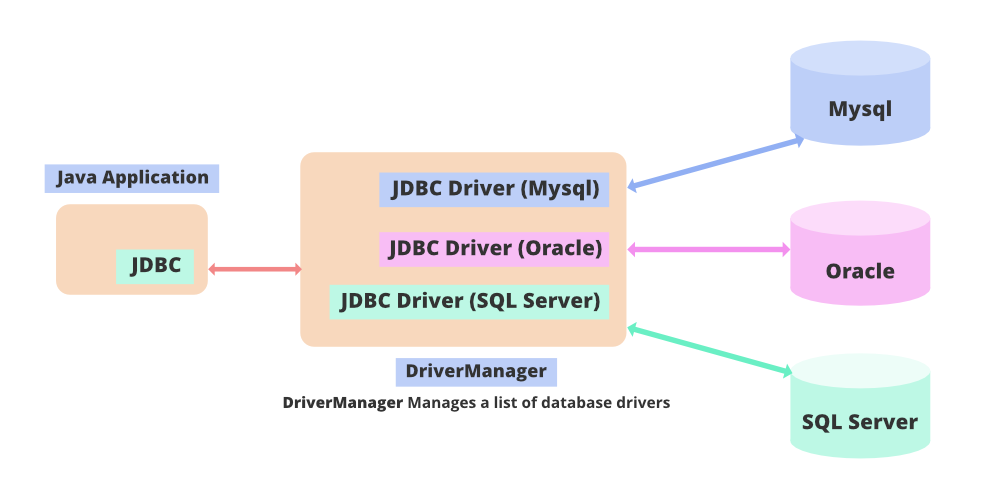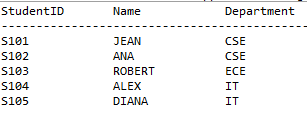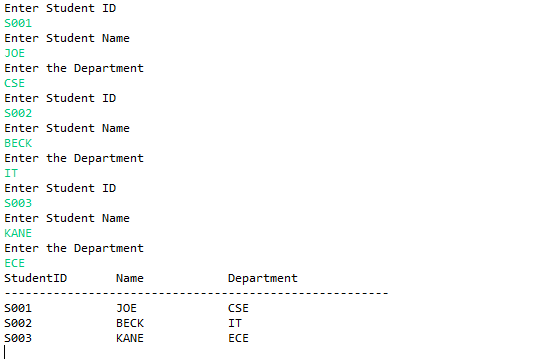# How to Execute Multiple SQL Commands on a Database Simultaneously in JDBC?

• Last Updated : 08 Jun, 2022

Java Database Connectivity also is known as JDBC is an application programming interface in Java that is used to establish connectivity between a Java application and database. JDBC commands can be used to perform SQL operations from the Java application. Demonstrating execution of multiple SQL commands on a database simultaneously using the addBatch() and executeBatch() commands of JDBC.The addBatch() command is used to queue the SQL statements and executeBatch() command is used to execute the queued SQL statements all at once. In order to use SQL statements in the Java application, ”java.sql” package needs to be imported in the beginning of the Java application. The Java application is connected to the database using the getConnection() method of DriverManager class. The getConnection() method takes three parameters URLs, username and password.

Goal: Demonstrates two examples of which one uses the Statement Interface and the other uses PreparedStatement Interface. The PreparedStatement performs better than the Statement interface. Statement interface can be used to execute static SQL queries whereas PreparedStatement interface is used to execute dynamic SQL queries multiple times.

Example 1: Using Statement Interface

In this example, the java.sql package classes and interfaces are imported. The Statement interface is used to execute the sql statements. The table is creation sql statement along with record insertion sql statement are added to the batch using the addBatch() command. When all the statements are batched the executeBatch() command is executed which runs all the batched queries simultaneously. The sql statements may throw SQL Exceptions which must be handled in a try catch block to avoid abrupt termination of the program. After the table is created and records are inserted, to view the data in the table the select query is executed. The result obtained by executing the select query is stored in the ResultSet cursor. The cursor is iterated using the next() method and the records are displayed on the screen.

Implementation: Using the standard interface

## Java

 `// Step 1: Create a database``// SQL database imported``import` `java.sql.Connection;``import` `java.sql.DriverManager;``import` `java.sql.ResultSet;``import` `java.sql.Statement;` `public` `class` `BatchCommand {` `    ``// Main driver method``    ``public` `static` `void` `main(String args[])``    ``{` `        ``// Try block to check if exception occurs``        ``try` `{` `            ``// Step 2: Loading driver class``            ``// Using forName()``            ``Class.forName(``"oracle.jdbc.OracleDriver"``);` `            ``// Step 3: Create connection object``            ``Connection con = DriverManager.getConnection(``                ``"jdbc:oracle:thin:@localhost:1521:xe"``,``                ``"username"``, ``"password"``);``            ``Statement s = con.createStatement();``          ` `            ``// Step 4: Create a statement / create table``            ``String sql1``                ``= ``"CREATE TABLE STUDENT(STUDENTID VARCHAR2(10) PRIMARY KEY,NAME VARCHAR2(20),DEPARTMENT VARCHAR2(10))"``;` `            ``// Step 5: Process a query``            ``// Insert records in the table``            ``String sql2``                ``= ``"INSERT INTO STUDENT VALUES('S101','JEAN','CSE')"``;``            ``String sql3``                ``= ``"INSERT INTO STUDENT VALUES('S102','ANA','CSE')"``;``            ``String sql4``                ``= ``"INSERT INTO STUDENT VALUES('S103','ROBERT','ECE')"``;``            ``String sql5``                ``= ``"INSERT INTO STUDENT VALUES('S104','ALEX','IT')"``;``            ``String sql6``                ``= ``"INSERT INTO STUDENT VALUES('S105','DIANA','IT')"``;``            ``s.addBatch(sql1);``            ``s.addBatch(sql2);``            ``s.addBatch(sql3);``            ``s.addBatch(sql4);``            ``s.addBatch(sql5);``            ``s.addBatch(sql6);` `            ``// Step 6: Process the results``            ``// execute the sql statements``            ``s.executeBatch();``            ``ResultSet rs``                ``= s.executeQuery(``"Select * from Student"``);` `            ``// Print commands``            ``System.out.println(``                ``"StudentID\tName\t\tDepartment"``);``            ``System.out.println(``                ``"-------------------------------------------------------"``);` `            ``// Condition to check pointer pointing``            ``while` `(rs.next()) {``                ``System.out.println(rs.getString(``1``) + ``"\t\t"``                                   ``+ rs.getString(``2``)``                                   ``+ ``"\t\t"``                                   ``+ rs.getString(``3``));``            ``}` `            ``// Step 7: Close the connection``            ``con.commit();``            ``con.close();``        ``}` `        ``// Catch block to handle exceptions``        ``catch` `(Exception e) {` `            ``// Print line number if exception occurred``            ``System.out.println(e);``        ``}``    ``}``}`

SQL commands over database using addBatch() method with the involvement of executeBatch()

## Java

 `// Step 1: Importing database``// SQL database imported``import` `java.sql.Connection;``import` `java.sql.DriverManager;``import` `java.sql.ResultSet;``import` `java.sql.Statement;` `public` `class` `BatchCommand {` `    ``// Main driver method``    ``public` `static` `void` `main(String args[])``    ``{` `        ``// Try block to handle if exception occurs``        ``try` `{` `            ``// Step 2: loading driver class``            ``Class.forName(``"oracle.jdbc.OracleDriver"``);` `            ``// Step 3: create connection object``            ``Connection con = DriverManager.getConnection(``                ``"jdbc:oracle:thin:@localhost:1521:xe"``,``                ``"username"``, ``"password"``);``            ``Statement s = con.createStatement();` `            ``// Step 4: Create a statement``            ``// Create table``            ``String sql1``                ``= ``"CREATE TABLE STUDENT(STUDENTID VARCHAR2(10) PRIMARY KEY,NAME VARCHAR2(20),DEPARTMENT VARCHAR2(10))"``;` `            ``// Step 5: Execute a query``            ``// Insert records in the table``            ``String sql2``                ``= ``"INSERT INTO STUDENT VALUES('S101','JEAN','CSE')"``;``            ``String sql3``                ``= ``"INSERT INTO STUDENT VALUES('S102','ANA','CSE')"``;``            ``String sql4``                ``= ``"INSERT INTO STUDENT VALUES('S103','ROBERT','ECE')"``;``            ``String sql5``                ``= ``"INSERT INTO STUDENT VALUES('S104','ALEX','IT')"``;``            ``String sql6``                ``= ``"INSERT INTO STUDENT VALUES('S105','DIANA','IT')"``;``            ``s.addBatch(sql1);``            ``s.addBatch(sql2);``            ``s.addBatch(sql3);``            ``s.addBatch(sql4);``            ``s.addBatch(sql5);``            ``s.addBatch(sql6);` `            ``// Step 6: Process the statements``            ``// Create an int[] to hold returned values``            ``s.executeBatch();``            ``ResultSet rs``                ``= s.executeQuery(``"Select * from Student"``);` `            ``// Print statements``            ``System.out.println(``                ``"StudentID\tName\t\tDepartment"``);``            ``System.out.println(``                ``"-------------------------------------------------------"``);` `            ``// Condition check for pointer pointing which``            ``// record``            ``while` `(rs.next()) {``                ``System.out.println(rs.getString(``1``) + ``"\t\t"``                                   ``+ rs.getString(``2``)``                                   ``+ ``"\t\t"``                                   ``+ rs.getString(``3``));``            ``}` `            ``// Step 7: Close the connection``            ``con.commit();``            ``con.close();``        ``}` `        ``// Catch block to handle exception``        ``catch` `(Exception e) {` `            ``// Print line number where exception occurred``            ``System.out.println(e);``        ``}``    ``}``}`

OutputExample 2: In this example, the java.sql package classes and interfaces are imported. The PreparedStatement interface is used to execute the SQL statements. The table is the creation SQL statement along with record insertion SQL statement are added to the batch using the addBatch() command. When all the statements are batched the executeBatch() command is executed which runs all the batched queries simultaneously. The sql statements may throw SQL Exceptions which must be handled in a try-catch block to avoid abrupt termination of the program. After the table is created and records are inserted, to view the data in the table the select query is executed. The result obtained by executing the select query is stored in the ResultSet cursor. The cursor is iterated using the next() method and the records are displayed on the screen. Unlike the previous example, it takes dynamic input from the user. Hence, using the PreparedStatement has performance benefits.

Code Implementation

## Java

 `import` `java.sql.Connection;``import` `java.sql.DriverManager;``import` `java.sql.PreparedStatement;``import` `java.sql.ResultSet;``import` `java.sql.Statement;``import` `java.util.*;``public` `class` `AddBatchCommand {``    ``public` `static` `void` `main(String args[])``    ``{``        ``Scanner scan = ``new` `Scanner(System.in);``        ``try` `{` `            ``// loading driver class``            ``Class.forName(``"oracle.jdbc.OracleDriver"``);` `            ``// create connection object``            ``Connection con = DriverManager.getConnection(``                ``"jdbc:oracle:thin:@localhost:1521:xe"``,``                ``"username"``, ``"password"``);` `            ``// create the table``            ``String sql1``                ``= ``"CREATE TABLE STUDENTS(STUDENTID VARCHAR2(10) PRIMARY KEY,NAME VARCHAR2(20),DEPARTMENT VARCHAR2(10))"``;``            ``PreparedStatement ps``                ``= con.prepareStatement(sql1);``            ``ps.execute(sql1);` `            ``// inserting records``            ``String sql``                ``= ``"Insert into Students values(?,?,?)"``;``            ``PreparedStatement ps1``                ``= con.prepareStatement(sql);``            ``for` `(``int` `i = ``0``; i < ``3``; i++) {``                ``System.out.println(``"Enter Student ID"``);``                ``String id = scan.nextLine();``                ``System.out.println(``"Enter Student Name"``);``                ``String name = scan.nextLine();``                ``System.out.println(``"Enter the Department"``);``                ``String dept = scan.nextLine();``                ``ps1.setString(``1``, id);``                ``ps1.setString(``2``, name);``                ``ps1.setString(``3``, dept);``                ``// adding to batch``                ``ps1.addBatch();``            ``}``            ``// executing the batch``            ``ps1.executeBatch();``          ` `            ``// viewing the table``            ``ResultSet rs``                ``= ps.executeQuery(``"Select * from Students"``);``            ``System.out.println(``                ``"StudentID\tName\t\tDepartment"``);``            ``System.out.println(``                ``"-------------------------------------------------------"``);``            ``while` `(rs.next()) {``                ``System.out.println(rs.getString(``1``) + ``"\t\t"``                                   ``+ rs.getString(``2``)``                                   ``+ ``"\t\t"``                                   ``+ rs.getString(``3``));``            ``}``            ``con.commit();``            ``con.close();``        ``}` `        ``catch` `(Exception e) {``            ``System.out.println(e);``        ``}``    ``}``}`

Output: Illustrating multiple SQL commands on a database simultaneously:My Personal Notes arrow_drop_up